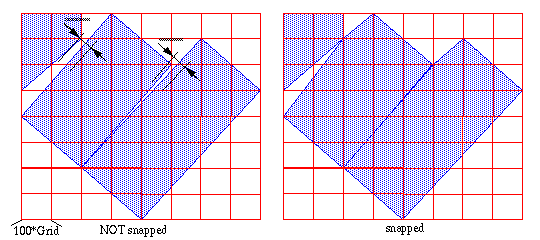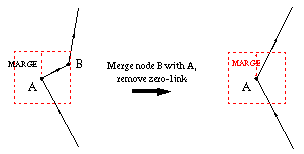Prepare the GRAPH

The following actions makes sure that the total graph (containing all polygons), is suitable for performing a boolean operation.

## convert the polygons into graphs

All polygons are converted into graphs and put into a list of graphs (graphlist). See graphs structure
Assuming the input data is supplied in doubles, the coordinates will be converted to 64bit integers.
This is done using two scale factors, DGRID and GRID.
DGRID defines the accuracy  the user wants
an accuracy of two digits means DGRID should be set to  DGRID = 0.01

Although in the boolean algorithm the accuracy is higher, the end result will be rounded to the DGRID.

GRID defines the number of digits the boolean algorithm will use to calculate extra intersection.
If for instance two segments intersect the intersection point does not have to be on the GRID.
If the GRID would be set to 1, there would be no integer number in between to define this intersection point.
With the GRID set to 100 there is place for those intersections, in this case the intersection point would be (50,50).

In fact the algorithm uses a kind of integer fixed point calculation for coordinates, the fractional part is always fixed (least significant 2 digits) .

For instance if the DGRID = 0.01 and GRID = 100 and the original polygon point is (1234.56,4567.89).
The following coordinate will be used inside the boolean algorithms  (12345600,45678900).
So multiplied by 1/DGRID converts the doubles to integers.
GRID = 100  means multiply those integers with an extra factor to create space for the boolean algorithm to create intersections in between.
In the end all points will be converted back to double with an accuracy of DGRID.
This means all new intersection points will also be rounded to the DGRID in the end, if the user wants those intersection to be more accurate
than his input data, the DGRID should be set smaller than the accuracy of the input data.

## Round

The boolean algorithm works internal with "64 bit integers", all the coordinates need to be rounded to a GRID.
Currently the GRID is set to 100. This means that all input coordinates will be rounded at  two decimals.
Since every coordinate is rounded to GRID, the minimum length of a segment in the input data can be one GRID (zero length segments are removed). Diagonal the minimum length will be "sqrt(GRID)". Two such segments can still intersect since GRID is set two 100.

#### minimum length segments#### MARGE

What is defined as small is set by the factor MARGE, which can be set by the user. MARGE is heavely used all through the algorithm. It takes care of preventing glitches, because it makes nodes snap to lines and other nodes. So it can be seen as a snapfactor. It is important to understand that the snapping is not the same as rounding to a GRID. Many graphical drawing tools work with a GRID, even without knowing it the user will introduce glitches into his data. Next to that, many graphical interface formats super impose another GRID on top of the data, resulting in even bigger glitches. The MARGE factor takes care of such unwanted effects. In remove glitches. gives an example, it may look that the drawing is heavely changed by this action, but since the MARGE is normally small compared to the size of the polygon itself, this is not really the case. It does not make much sence to set MARGE smaller than the GRID, since everything is rounded to GRID first. Normally the GRID is set much smaller than MARGE. Even if MARGE takes care of snapping vertexes, in the end every intersection wil be rounded to the GRID again. This is not a problem, because snapping problems are solved at that stage.
Assume the following settings for DGRID, GRID and  MARGE:

DGRID      = 0.01;
MARGE     = 0.1;

Those factors are all based on the points that are put in the original polygons using doubles for the vertexes.
The coordinates and factors used by the boolean algorithm are scaled properly, before doing an operation.
MARGE = 0.1 results in snapping/breaking segments to reach another vertex when the distance to this vertex is smaller than 0.1.

Just before converting the polygons into graphs the factors will be set as follows:

DGRID      = 1/DGRID;
MARGE     = MARGE*GRID*DGRID;

With DGRID=0.01 , GRID=100, MARGE=0.1 this would result in MARGE=1000 that is used inside the boolean algorithm.
After the boolean operation, they will be set back to the original value.

#### remove glitches## simplify

Simplify works on the input data, it makes sure that the input data does not contain segments that are not needed and could cause problems.
Simplify means removing redundant coordinates, for instance the segment coordinates are at the same position. This would result in a zero length segment, which is impossible to handle because its direction is not defined.
Also very small segments will be removed. What is small is defined by the factor MARGE.
In removing zero-links. node B is removed since it is within MARGE distance to node A.